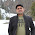### Map.merge() - One method to rule them allSuwalszczyzna
Russian translation available: Map.merge () - метод, чтобы управлять всеми остальными

I don’t often explain a single method in JDK, but when I do, it’s about `Map.merge()`. Probably the most versatile operation in the key-value universe. And also rather obscure and rarely used. `merge()` can be explained as follows: it either puts new value under the given key (if absent) or updates existing key with a given value (UPSERT). Let’s start with the most basic example: counting unique word occurrences. Pre-Java 8 (read: pre-2014!) code was quite messy and the essence was lost in implementation details:

```var map = new HashMap<String, Integer>();
words.forEach(word -> {
var prev = map.get(word);
if (prev == null) {
map.put(word, 1);
} else {
map.put(word, prev + 1);
}
});```
However, it works and for given input produces desired output:

```var words = List.of("Foo", "Bar", "Foo", "Buzz", "Foo", "Buzz", "Fizz", "Fizz");
//...
{Bar=1, Fizz=2, Foo=3, Buzz=2}```
OK, but let’s try to refactor it to avoid conditional logic:

```words.forEach(word -> {
map.putIfAbsent(word, 0);
map.put(word, map.get(word) + 1);
});```
That’s nice! `putIfAbsent()` is a necessary evil, otherwise, the code breaks on the first occurrence of a previously unknown word. Also, I find `map.get(word)` inside `map.put()` a bit awkward. Let’s get rid of it as well!

```words.forEach(word -> {
map.putIfAbsent(word, 0);
map.computeIfPresent(word, (w, prev) -> prev + 1);
});```
`computeIfPresent()` invokes given transformation only if key in question (`word`) exists. Otherwise does nothing. We made sure key exists, by initializing it to zero, so incrementation always works. Can we do better? We can cut the extra initialization, but I wouldn’t recommend it:

```words.forEach(word ->
map.compute(word, (w, prev) -> prev != null ? prev + 1 : 1)
);```
`compute()` is like `computeIfPresent()`, but invoked irrespective to the existence of given key. If value for the key does not exist, `prev` argument is `null`. Moving simple `if` to ternary expression hidden in lambda is far from optimal. This is where `merge()` operator shines. Before I’ll show you the final version, let’s see a slightly simplified default implementation of `Map.merge()`:

```default V merge(K key, V value, BiFunction<V, V, V> remappingFunction) {
V oldValue = get(key);
V newValue = (oldValue == null) ? value :
remappingFunction.apply(oldValue, value);
if (newValue == null) {
remove(key);
} else {
put(key, newValue);
}
return newValue;
}```
The code snippet is worth a thousand words. `merge()` works in two scenarios. If the given key is not present, it simply becomes `put(key, value)`. However, if said key already holds some value, our `remappingFunction` may merge (duh!) the old and the one. This function is free to:

• overwrite old value by simply returning the new one: `(old, new) -> new`
• keep the old value by simply returning the old one: `(old, new) -> old`
• somehow merge the two, e.g.: `(old, new) -> old + new`
• or even remove old value: `(old, new) -> null`
As you can see `merge()` is quite versatile. So how does our academic problem look like with `merge()`? It’s quite pleasing:

```words.forEach(word ->
map.merge(word, 1, (prev, one) -> prev + one)
);```
You can read it as follows: put `1` under `word` key if absent, otherwise add `1` to existing value. I named one of the parameters “`one`” because in our example it’s always… 1. Sadly `remappingFunction` takes two parameters, where the second one is the value we are about to upsert (insert or update). Technically we know this value already, so `(word, 1, prev -> prev + 1)` would be much easier to digest. But there’s no such API.

All right, but is `merge()` really useful? Imagine you have an account operation (constructor, getters and other useful properties omitted):

```class Operation {
private final String accNo;
private final BigDecimal amount;
}```
And a bunch of operations for different accounts:

```var operations = List.of(
new Operation("123", new BigDecimal("10")),
new Operation("456", new BigDecimal("1200")),
new Operation("123", new BigDecimal("-4")),
new Operation("123", new BigDecimal("8")),
new Operation("456", new BigDecimal("800")),
new Operation("456", new BigDecimal("-1500")),
new Operation("123", new BigDecimal("2")),
new Operation("123", new BigDecimal("-6.5")),
new Operation("456", new BigDecimal("-600"))
);```
We would like to compute balance (total over operations’ amounts) for each account. Without `merge()` this is quite cumbersome:

```var balances = new HashMap<String, BigDecimal>();

operations.forEach(op -> {
var key = op.getAccNo();
balances.putIfAbsent(key, BigDecimal.ZERO);
});```
But with a little help of `merge()`:

```operations.forEach(op ->
balances.merge(op.getAccNo(), op.getAmount(),
);```
Do you see a method reference opportunity here?

```operations.forEach(op ->
);```
I find this astoundingly readable. For each operation `add` given `amount` to given `accNo`. The results are as expected:

`{123=9.5, 456=-100}`

# `ConcurrentHashMap`

`Map.merge()` shines even brighter when you realize it’s properly implemented in `ConcurrentHashMap`. This means we can atomically perform insert-or-update operation. Single line and thread-safe. `ConcurrentHashMap` is obviously thread-safe, but not across many operations, e.g. `get()` and then `put()`. However `merge()` makes sure no updates are lost.

1.